# Primitive root

(diff) ← Older revision | Latest revision (diff) | Newer revision → (diff)

## Definition

Supposeis a natural number such that the multiplicative group modulo, i.e., the group, is a cyclic group. (This happens if and only ifis of one of these four forms: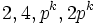, whereis a prime number and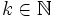. Then, a primitive root modulois a residue class modulothat generates the cyclic group.

We often use the term primitive root for an integer representative of such a residue class.

The number of primitive roots modulo, if the multiplicative group is cyclic, is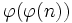whereis the Euler totient function. This is because the order of the multiplicative group is, and the number of generators of a cyclic group equals the Euler phi-function of its order.

For a proof of the characterization of natural numbers for which the multiplicative group is cyclic, see Classification of natural numbers for which the multiplicative group is cyclic.

## Particular cases

### Number of primitive roots

Here are the particular cases:

Value ofNumber of primitive roots
2 1
4 1(odd prime),odd,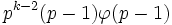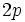,odd,odd,### Values of primitive roots

For an odd prime, any number that is a primitive root modulo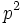continues to be a primitive root modulo higher powers of. We thus list primitive roots only for numbers of the formand.

Value ofNumber of primitive roots Smallest absolute value primitive root (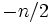to) Smallest positive primitive root All primitive roots fromto2 1 1 1 1
3 1 -1 2 2
4 1 -1 3 3
5 2 2,-2 2 2,3
7 2 -2 3 3,5
9 2 2 2 2,5
11 4 2 2 2,6,7,8
13 4 2 2 2,6,7,11
17 8 3,-3 3 3,5,6,7,10,11,12,14

## Relation with other properties

### Other related properties

• Quadratic nonresidue is a number relatively prime to the modulus that is not a square modulo the modulus. Any primitive root must be a quadratic nonresidue except in the case where the modulus is. This is because the multiplicative group has even order and hence its generator cannot be a square.

## Facts

### Known facts

For most primes, finding a primitive root is hard work. However, for those primeswherehas a very small list of prime factors, it is relatively easy to find a primitive root. Some cases are below:

Statement Applicable prime type Description in terms of factorization ofQuadratic nonresidue that is not minus one is primitive root for safe prime safe primeis twice of a prime. The prime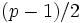is called a Sophie Germain prime.
Safe prime has plus or minus two as a primitive root safe primeis twice of a prime. The primeis called a Sophie Germain prime.
Quadratic nonresidue equals primitive root for Fermat prime Fermat prime power of 2. In fact, it is a power of a power of 2
Fermat prime greater than three implies three is primitive root Fermat prime power of 2. In fact, it is a power of a power of 2, and from the given condition, it is also 1 mod 4.

### Conjectures and conditionally known facts

Artin's conjecture on primitive roots states that any integer that is not a perfect square and that is not equal to -1 occurs as a primitive root for infinitely many primes. The conjecture is open, but partial results are known:

Name of conjecture/fact Statement Conditional to ...
Hooley's theorem Artin's conjecture holds for all(special cases of) generalized Riemann hypothesis
Gupta-Ram Murty theorem Artin's conjecture holds for infinitely manyUnconditional
Heath-Brown theorem on Artin's conjecture Artin's conjecture holds for all but two exceptional values of. However, no explicit information about the explicit values ofUnconditional